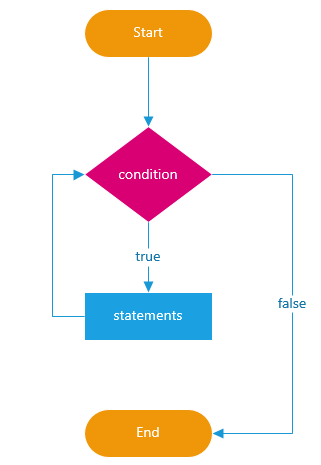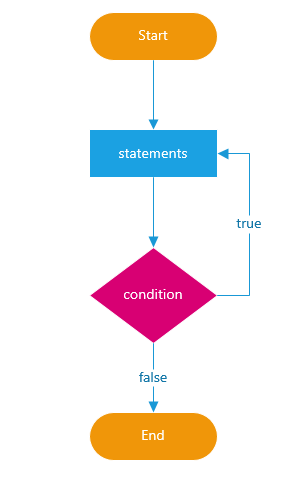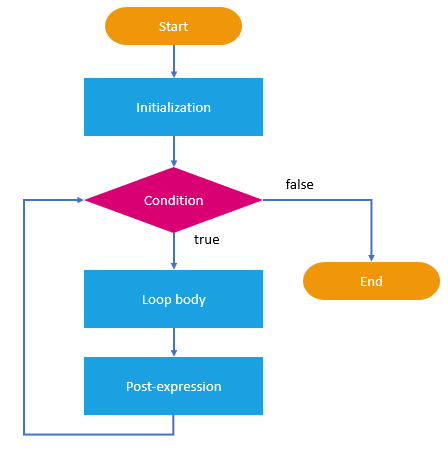# JavaScript Loop

## JavaScript Loop with Examples

In this article, I am going to discuss JavaScript Loop with Examples. Please read our previous article where we discussed JavaScript Conditional Statements in Detail. At the end of this article, you will understand what is JavaScript Loop and when and how to use Loop in JavaScript with examples.

##### What is JavaScript Loop?

Loop is a JavaScript control statement which executes blocks of code repeatedly. A code block or block of code is a code between {and}. Types of loops in JavaScript are:

• while loop
• do-while loop
• for loop
• for…in loop

Nested loops – a composition of loops

##### While Loop in JavaScript

The while loop executes a block of code repeatedly i.e. it execute the code block until the given condition is true else the loop gets terminated.

We use a variable to check the condition. The variable meets the condition at the entrance of the while loop. If the variable does not meet the condition then the loop will not get executed not even for a single time. Due to this, it is possible that statements inside the while loop is never executed. The following flowchart describes the while loop statement.##### Syntax of JavaScript While Loop:
```while(condition){
block of code to be executed
}```
##### Example of While Loop in JavaScript:

In the below code, the value of the counter variable is initially 0, the condition with the loop is while the counter variable is less than 10, the statement within the while block is going to be executed. Here, counter++ means counter=counter+1, so the counter will get incremented with 1 every time. As soon as the counter becomes 10 the loop gets terminated.

```<script type="text/javascript">
var counter = 0;
while (counter < 10) {
console.log('Number : ' + counter);
counter++;
}
</script>```
##### How does the while loop work in JavaScript?

The while loop in JavaScript works exactly in the same as the while loop works in other programming languages such as C, Java, C#, etc. as follows:

1. The While loop first check the condition
2. If the given condition is true, then the statement block within the while loop are executed
3. This process is going to be repeated as long as the condition evaluates to true.
4. Once the condition false, then loop terminates.
##### What is infinite loops?

If you forgot to update the variable which is evaluates in the condition, then it will create a never ending loop which is also called as an infinite loop. For example, if you notice in the below code, we have commented the line which updates the counter variable. As a result of this, the counter variable is always ZERO and the condition in the while loop is always going to be true. In other words, we can say that, the condition will never become false, and we have an infinite while loop.

```<script type="text/javascript">
var counter = 0;
while (counter < 10) {
console.log('Number : ' + counter);
//counter++;
}
</script>```
##### How to solve the problem?

We can solve the problem by using break statement within the while loop in JavaScript.

##### The break statement in JavaScript

As we have seen in the earlier chapter of JavaScript Condition for switch statements where the break statement is used. There it was used to “come out” of the switch statements. The break statement can also be used in the loop to “comes out” of the loop by exiting the inner-most loop where it is declared.

As the name suggests the break statement is used in JavaScript to break or terminate the loop in between and continues executing the code placed after the loop if there is any.

##### Example to understand JavaScript break statement:

Let us understand the use of break statement in a while loop with an example. Now we want the while loop to terminate or break when the counter variable becomes 5. If you notice in the below code, we use a if statement to check the counter variable and when the counter variable becomes 5, the if condition becomes true and this will execute the break statement, which will terminate the execution of the while loop.

```<script type="text/javascript">
var counter = 0;
while (counter < 10) {
if (counter == 5)
break;//break loop only if counter==5
console.log('Number : ' + counter);
counter++;
}
</script>```

Output:

Number: 0
Number: 1
Number: 2
Number: 3
Number: 4

##### The Continue Statement in JavaScript

The continue statements will break the current loop where it is defined and continues with the next iteration in the loop. In others words we can say that, the continue statement tells the JavaScript interpreter to skip remaining code that is present after this statement and start the next iteration of the loop.

##### Example to understand the JavaScript Continue Statement:

Let us understand the use of JavaScript Continue statement with an example. The following example prints all the numbers between 1 and 10 except 5. In this case, when the counter variable becomes 5, the if condition becomes true, which cause the continue statement to execute.

```<script type="text/javascript">
var counter = 0;
while (counter < 10) {
counter++;
if (counter == 5)
continue;//skip the code in loop only if counter==5
console.log('Number : ' + counter);
}
</script>```

Output:
Number: 1
Number: 2
Number: 3
Number: 4
Number: 6
Number: 7
Number: 8
Number: 9
Number: 10

##### The Label Statement in JavaScript:

In JavaScript, we can label a statement for using it later. The label can be any valid identifier.

Syntax: label: statement;

We can reference the label by using the break or continue statement. Typically, we use the label with a nested loop such as for, do-while, and while loop.

Example to understand the JavaScript label statement:

```outer: for (let i = 0; i <= 5; i++) {
console.log(i);
}```

Above example labels the loop using the outer label.

##### Using label with break and continue statement

Syntax:
break labelname;
continue labelname;

##### Using JavaScript break with label

Example:

```<script type="text/javascript">
var counter = 0;
check: while (counter < 10) {
if (counter == 5)
break check;//break loop only if counter==5
console.log('Number : ' + counter);
counter++;
}
</script>```
##### Using JavaScript continue with label

Example:

```<script type="text/javascript">
var counter = 0;
skiptest: while (counter < 10) {
if (counter == 5)
continue skiptest;//skip the code in loop only if counter==5
console.log('Number : ' + counter);
counter++;
}
</script>```
##### Do-while Loop in JavaScript

The do-while loop executes the block of code repeatedly i.e. in a loop until the given condition is found true else the loop gets terminated.

The difference between do while and while loop is that even the condition is found false, the do while loop executes the block of code ONCE, and then it will repeat the loop until the specified condition is true. Whereas while loop gets terminated at the initial stage if the condition is false. The reason for this is, while loop checks the condition at the beginning, where as do…while… loop checks the condition at the end of the loop

Note: If we want to execute the statement or code at least once and check the condition after each iteration, then you should use the do-while loop.

The following flowchart describes the do-while loop statement:##### Syntax:
```do {
block of code to be executed
}
while(condition);```
##### Example:
```<script type="text/javascript">
var i = 0;
do {
document.write(i + " ");
i++; // incrementing i by 1
} while (i < 5);
</script>```
##### JavaScript for Loop

The for loop repeats the elements for the fixed number of times. It is used when we already know how many times the script should run. The following flowchart describes the for loop:##### Syntax:
```for (initialization; condition; increment/decrement) {
// block of code to be executed--body
}```

This loop contains the initial value, condition, increment/decrement value in a single line, which in on other looping are used in a separate line, the scope of a for loop is inside the curly brackets used with it.

Initialization: The initialization expression initializes the loop. It is executed once, just before the execution of the block of code

Condition: The condition is an expression that is checked before each iteration of the loop (based on loop condition)

Increment/decrement: The increment/decrement segments are executed every time after the block of code has been executed, till the condition remains true.

Body: The code that will be executed at each iteration

##### Example:
```<script type="text/javascript">
for (var number = 0; number < 10; number++) {
console.log(number);
}
</script>```
##### Complex for Loop

Complex for loop may have several counter variables

##### Example:
```<script type="text/javascript">
for (var i = 1, sum = 1; i <= 128; i = i * 2, sum += i) {
console.log('i = ' + i + ', sum = ' + sum);
}
</script>```

Output:
i = 1, sum = 1
i = 2, sum = 3
i = 4, sum = 7
i = 8, sum = 15

##### for…in Loop in JavaScript

The JavaScript for…in Loop statement iterates the properties of an object. This loop is used with arrays and objects, to access their elements and properties respectively.

• For arrays / strings iterates over their indexes (0length-1)
• For any other object, for-in iterates over its properties

In for..in looping the variable value starts from zero and increases itself with one until it reach to the length of array.

##### Syntax:
```for (variablename in object) {
statement or block to execute
}```
##### Iterating over the elements of an array / string

Example:

```<script type="text/javascript">
var arr = [10, 20, 30, 40, 50];
for (var index in arr) { console.log(arr[index]) }// 10, 20, 30, 40, 50

var str = "welcome";
for (var index in str) { console.log(str[index]) }// w, e, l, c, o, m, e
</script>```
##### Iterating over the properties of an object:

Example:

```<script type="text/javascript">
var obj = { name: 'Steve', age: 23, location: 'Sofia' };
for (var key in obj) { console.log(obj[key]); }// Steve, 23 , Sofia
</script>```
##### Nested Loop

Nested loop is a loop inside another loop

Syntax:

```for (initialization; condition; increment/decrement) {
for (initialization; condition; increment/decrement) {
statements;
}
…
}```